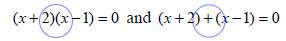### Home > CAAC > Chapter 8 > Lesson 8.2.3 > Problem8-65

8-65.

Compare the two equations below. Homework Help ✎

$(x+2)(x-1)=0$ and $(x+2)+(x-1)=0$

1. How are the equations different?In the first equation, the polynomials are multiplied. In the second equation, they are added.

1. Solve both equations.

Set each factor equal to zero and solve.

For the second equation, combine like terms.